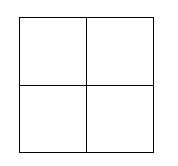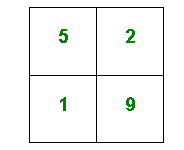#### You may also like### Pebbles

Place four pebbles on the sand in the form of a square. Keep adding as few pebbles as necessary to double the area. How many extra pebbles are added each time?### Bracelets

Investigate the different shaped bracelets you could make from 18 different spherical beads. How do they compare if you use 24 beads?### Sweets in a Box

How many different shaped boxes can you design for 36 sweets in one layer? Can you arrange the sweets so that no sweets of the same colour are next to each other in any direction?

# Reach 100

##### Age 7 to 11Challenge Level

Here is a grid of four "boxes":You must choose four different digits from $1 - 9$ and put one in each box. For example:This gives four two-digit numbers:

 $52$ (reading along the $1$st row) $19$ (reading along the $2$nd row) $51$ (reading down the left hand column) $29$ (reading down the right hand column)

In this case their sum is $151$.

Try a few examples of your own.
Is there a quick way to tell if the total is going to be even or odd?

Your challenge is to find four different digits that give four two-digit numbers which add to a total of $100$.

How many ways can you find of doing it?

You may be interested in the other problems in our Mastering Mathematics: Developing Generalising and Proof Feature.

This problem is adapted from Make 200 from 'Mathematical Challenges for Able Pupils Key Stages 1 and 2', published by DfES.